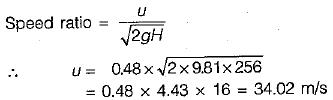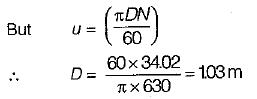Courses

# Fluid Machines - 1

## 10 Questions MCQ Test Mock Test Series for Civil Engineering (CE) GATE 2020 | Fluid Machines - 1

Description
This mock test of Fluid Machines - 1 for Civil Engineering (CE) helps you for every Civil Engineering (CE) entrance exam. This contains 10 Multiple Choice Questions for Civil Engineering (CE) Fluid Machines - 1 (mcq) to study with solutions a complete question bank. The solved questions answers in this Fluid Machines - 1 quiz give you a good mix of easy questions and tough questions. Civil Engineering (CE) students definitely take this Fluid Machines - 1 exercise for a better result in the exam. You can find other Fluid Machines - 1 extra questions, long questions & short questions for Civil Engineering (CE) on EduRev as well by searching above.
QUESTION: 1

### A pump running at 1414 rpm delivers 256 lps of water against a head of 16 m. The pump is of the

Solution: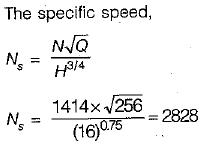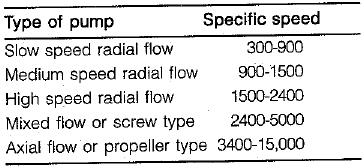QUESTION: 2

### Consider the following statements: 1. Specific speed uniquely determines the type, shape and design of the turbine. 2. Both unit speed ‘n11’ and unit discharge ‘Q11’ are necessary to uniquely determine the type and design of the turbine. Which of these statements is/are correct in respect of a hydraulic turbine?

Solution:

Specific speed is independent of dimension or size of the actual turbine and specific turbineTherefore besides unit speed and unit discharge overall efficiency is also required to determine type and design of turbine.

QUESTION: 3

### If the radius of the centrifugal pump impeller is reduced from 10 cm, the head developed by the pump will change from 10 m to

Solution: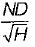will remain same even when diameter is reduced.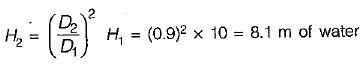QUESTION: 4

A Francis turbine working at 400 rpm has a unit speed of 50 rpm and develops 500 kW of power. What is the effective head under which this turbine operates?

Solution:

Unit speed of the turbine is given by,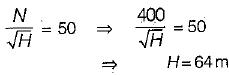QUESTION: 5

For a hydro-electric project with reaction turbine, the draft tube at the exit from the turbine is

Solution:

Draft tube must be air-tight and under all-conditions of its operation its lower end must be submerged below the level of water in the tail race. It has two purposes:
(i) Permits a negative or suction head to be established at the runner exit.
(ii) Converts a large portion of velocity energy rejected from the runner into useful pressure energy.

QUESTION: 6

In case of semi-circular vanes, thb theoretical maximum efficiency of the wheel can be

Solution: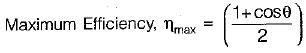For θ = 0°, the curved vanes will become semicircular and ηmax = 1 or 100%.

QUESTION: 7

Two geometrically similar pumps are running at the same speed of 100 rpm and lifting water against the heads of 25 m and 16 m respectively. First pump is having an impeller diameter of 300 mm. The impeller diameter of second pump shall be

Solution: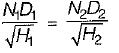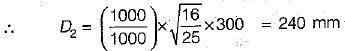QUESTION: 8

Which one of the following statements is correct?

Solution: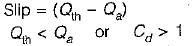If coefficient of discharge is more than one, negative slip would occur.

QUESTION: 9

The specific speed of a turbine under a head of 150 m to develop 2000 HP while running at 300 rpm is

Solution: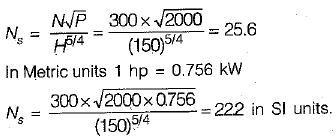QUESTION: 10

A pelton wheel operates at 630 rpm taking 3 m3/s of water under a head of 256 m with a speed ratio of 0.48 (Given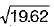= 4.43) What is the diameter of the impeller?

Solution: